Updating search results...# Calculus Based Physics

8 affiliated resources

# Search Resources

View
Selected filters: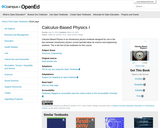Conditional Remix & Share Permitted
CC BY-SA
Rating
0.0 stars

Calculus-Based Physics is an introductory physics textbook designed for use in the two-semester introductory physics course typically taken by science and engineering students.

1 Mathematical Prelude
2 Conservation of Mechanical Energy I: Kinetic Energy & Gravitational Potential Energy
3 Conservation of Mechanical Energy II: Springs, Rotational Kinetic Energy
4 Conservation of Momentum
5 Conservation of Angular Momentum
6 One-Dimensional Motion (Motion Along a Line): Definitions and Mathematics
7 One-Dimensional Motion: The Constant Acceleration Equations
8 One-Dimensional Motion: Collision Type II
9 One-Dimensional Motion Graphs
10 Constant Acceleration Problems in Two Dimensions
11 Relative Velocity
12 Gravitational Force Near the Surface of the Earth, First Brush with Newton's 2nd Law
13 Freefall, a.k.a. Projectile Motion
14 Newton's Laws #1: Using Free Body Diagrams
15 Newton's Laws #2: Kinds of Forces, Creating Free Body Diagrams
16 Newton's Laws #3: Components, Friction, Ramps, Pulleys, and Strings
17 The Universal Law of Gravitation
18 Circular Motion: Centripetal Acceleration
19 Rotational Motion Variables, Tangential Acceleration, Constant Angular Acceleration
20 Torque & Circular Motion
21 Vectors: The Cross Product & Torque
22 Center of Mass, Moment of Inertia
23 Statics
24 Work and Energy
25 Potential Energy, Conservation of Energy, Power
26 Impulse and Momentum
27 Oscillations: Introduction, Mass on a Spring
28 Oscillations: The Simple Pendulum, Energy in Simple Harmonic Motion
29 Waves: Characteristics, Types, Energy
30 Wave Function, Interference, Standing Waves
31 Strings, Air Columns
32 Beats, The Doppler Effect
33 Fluids: Pressure, Density, Archimedes' Principle
34 Pascal's Principle, the Continuity Equation, and Bernoulli's Principle
35 Temperature, Internal Energy, Heat, and Specific Heat Capacity
36 Heat: Phase Changes
37 The First Law of Thermodynamics

Reviews available here: https://open.umn.edu/opentextbooks/textbooks/calculus-based-physics-i

Subject:
Physical Science
Physics
Material Type:
Textbook
Provider:
BCcampus
Provider Set:
BCcampus Faculty Reviewed Open Textbooks
Author:
Jeffrey W. Schnick
10/28/2014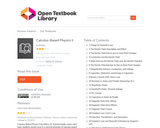Conditional Remix & Share Permitted
CC BY-SA
Rating
0.0 stars

Calculus-Based Physics is an introductory physics textbook designed for use in the two-semester introductory physics course typically taken by science and engineering students.

1 Charge & Coulomb's Law
2 The Electric Field: Description and Effect
3 The Electric Field Due to one or more Point Charges
4 Conductors and the Electric Field
5 Work Done by the Electric Field, and, the Electric Potential
6 The Electric Potential Due to One or More Point Charges
7 Equipotential Surfaces, Conductors, and Voltage
8 Capacitors, Dielectrics, and Energy in Capacitors
9 Electric Current, EMF, Ohm's Law
10 Resistors in Series and Parallel; Measuring I & V
11 Resistivity, Power
12 Kirchhoff's Rules, Terminal Voltage
13 RC Circuits
14 Capacitors in Series & Parallel
15 Magnetic Field Intro: Effects
16 Magnetic Field: More Effects
17 Magnetic Field: Causes
19 Induction, Transformers, and Generators
20 Faraday's Law and Maxwell's Extension to Ampere's Law
21 The Nature of Electromagnetic Waves
22 Huygens's Principle and 2-Slit Interference
23 Single-Slit Diffraction
24 Thin Film Interference
25 Polarization
26 Geometric Optics, Reflection
27 Refraction, Dispersion, Internal Reflection
28 Thin Lenses: Ray Tracing
29 Thin Lenses: Lens Equation, Optical Power
30 The Electric Field Due to a Continuous Distribution of Charge on a Line
31 The Electric Potential due to a Continuous Charge Distribution
32 Calculating the Electric Field from the Electric Potential
33 Gauss's Law
34 Gauss's Law Example
35 Gauss's Law for the Magnetic Field, and, Ampere's Law Revisited
36 The Biot-Savart Law
37 Maxwell's Equations

Subject:
Physical Science
Physics
Material Type:
Textbook
Author:
Jeffrey Schnick
06/23/2020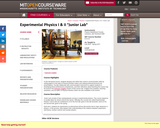Conditional Remix & Share Permitted
CC BY-NC-SA
Rating
0.0 stars

Junior Lab consists of two undergraduate courses in experimental physics. The courses are offered by the MIT Physics Department, and are usually taken by Juniors (hence the name). Officially, the courses are called Experimental Physics I and II and are numbered 8.13 for the first half, given in the fall semester, and 8.14 for the second half, given in the spring.The purposes of Junior Lab are to give students hands-on experience with some of the experimental basis of modern physics and, in the process, to deepen their understanding of the relations between experiment and theory, mostly in atomic and nuclear physics. Each term, students choose 5 different experiments from a list of 21 total labs.

Subject:
Physical Science
Physics
Material Type:
Full Course
Provider:
M.I.T.
Provider Set:
M.I.T. OpenCourseWare
Author:
Lecturers
Physics Department Faculty
and Technical Staff
01/01/2007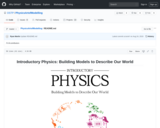Conditional Remix & Share Permitted
CC BY-SA
Rating
0.0 stars

This is an open-access textbook for calculus-based introductory physics courses. Anyone that complies with the license is welcome to modify and use this work for their own use, and we hope that you will choose to contribute. The textbook is specifically intended for a flipped-classroom approach, wherein students complete readings at home and the material is then discussed in class. The textbook thus contains questions and activities to engage readers. This text also includes a curriculum in experimental physics, detailing the scientific method and process, suggesting experiments to perform at home and in the lab, and has chapters that cover: writing and reviewing proposals, writing and reviewing reports, analyzing data, as well as an introduction to python. Finally, this textbook was written with many contributions from students! We hope that you may find it useful, and we are interested to know if you are using it!

The scientific method and physics
Comparing model and experiment
Describing motion in one dimension
Describing motion in multiple dimensions
Newton's Laws
Applying Newton's Laws
Work and energy
Potential energy and conservation of energy
Gravity
Linear momentum and the centre of mass
Rotational dynamics
Rotational energy and momentum
Simple harmonic motion
Waves
Fluid mechanics
Electric charges and fields
Gauss' Law
Electric potential
Electric current
Electric circuits
The magnetic force
Sources of magnetic field
Electromagnetic induction
The theory of Special Relativity
Appendix A: Vectors
Appendix B: Calculus
Appendix C: Guidelines for lab related activities
Appendix D: The python programming language

Subject:
Physical Science
Physics
Material Type:
Textbook
Author:
Emma Neary
Joshua Rinaldo
Olivia Woodman
Ryan D. Martin
10/08/2020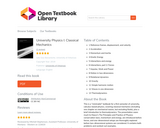Conditional Remix & Share Permitted
CC BY-NC
Rating
0.0 stars

This is a “minimalist” textbook for a first semester of university, calculus-based physics, covering classical mechanics (including one chapter on mechanical waves, but excluding fluids), plus a brief introduction to thermodynamics. The presentation owes much to Mazur’s The Principles and Practice of Physics: conservation laws, momentum and energy, are introduced before forces, and one-dimensional setups are thoroughly explored before two-dimensional systems are considered. It contains both problems and worked-out examples.

This is a “minimalist” textbook for a first semester of university, calculus-based physics, covering classical mechanics (including one chapter on mechanical waves, but excluding fluids), plus a brief introduction to thermodynamics. The presentation owes much to Mazur’s The Principles and Practice of Physics: conservation laws, momentum and energy, are introduced before forces, and one-dimensional setups are thoroughly explored before two-dimensional systems are considered. It contains both problems and worked-out examples.

Author
Julio Gea-Banacloche, University of Arkansas, Fayetteville

Subject:
Physical Science
Physics
Material Type:
Textbook
Author:
Julio Gea-Banacloche
06/23/2020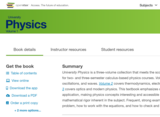Unrestricted Use
CC BY
Rating
0.0 stars

University Physics is a three-volume collection that meets the scope and sequence requirements for two- and three-semester calculus-based physics courses. Volume 1 covers mechanics, sound, oscillations, and waves. Volume 2 covers thermodynamics, electricity and magnetism, and Volume 3 covers optics and modern physics. This textbook emphasizes connections between between theory and application, making physics concepts interesting and accessible to students while maintaining the mathematical rigor inherent in the subject. Frequent, strong examples focus on how to approach a problem, how to work with the equations, and how to check and generalize the result.

Preface
Unit 1. Mechanics

Chapter 1: Units and Measurement
Chapter 2: Vectors
Chapter 3: Motion Along a Straight Line
Chapter 4: Motion in Two and Three Dimensions
Chapter 5: Newton's Laws of Motion
Chapter 6: Applications of Newton's Laws
Chapter 7: Work and Kinetic Energy
Chapter 8: Potential Energy and Conservation of Energy
Chapter 9: Linear Momentum and Collisions
Chapter 10: Fixed-Axis Rotation
Chapter 11: Angular Momentum
Chapter 12: Static Equilibrium and Elasticity
Chapter 13: Gravitation
Chapter 14: Fluid Mechanics
Unit 2. Waves and Acoustics

Chapter 15: Oscillations
Chapter 16: Waves
Chapter 17: Sound
Appendix A: Units
Appendix B: Conversion Factors
Appendix C: Fundamental Constants
Appendix D: Astronomical Data
Appendix E: Mathematical Formulas
Appendix F: Chemistry
Appendix G: The Greek Alphabet
Index

Subject:
Physical Science
Physics
Material Type:
Textbook
Provider:
Rice University
Provider Set:
OpenStax College
Author:
Alice Kolakowska
Bill Moebs
Daniel Bowman
David Anderson
David Smith
Dedra Demaree
Edward S. Ginsberg
Gerald Friedman
Joseph Trout
Kenneth Podolak
Kevin Wheelock
Lee LaRue
Lev Gasparov
Mark Lattery
Patrick Motl
Richard Ludlow
Samuel J. Ling
Takashi Sato
Tao Pang
08/03/2016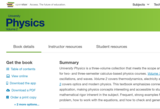Unrestricted Use
CC BY
Rating
0.0 stars

University Physics is a three-volume collection that meets the scope and sequence requirements for two- and three-semester calculus-based physics courses. Volume 1 covers mechanics, sound, oscillations, and waves. Volume 2 covers thermodynamics, electricity and magnetism, and Volume 3 covers optics and modern physics. This textbook emphasizes connections between theory and application, making physics concepts interesting and accessible to students while maintaining the mathematical rigor inherent in the subject. Frequent, strong examples focus on how to approach a problem, how to work with the equations, and how to check and generalize the result.

Unit 1: Thermodynamics

Chapter 1: Temperature and Heat
Chapter 2: The Kinetic Theory of Gases
Chapter 3: The First Law of Thermodynamics
Chapter 4: The Second Law of Thermodynamics
Unit 2: Electricity and Magnetism

Chapter 5: Electric Charges and Fields
Chapter 6: Gauss's Law
Chapter 7: Electric Potential
Chapter 8: Capacitance
Chapter 9: Current and Resistance
Chapter 10: Direct-Current Circuits
Chapter 11: Magnetic Forces and Fields
Chapter 12: Sources of Magnetic Fields
Chapter 13: Electromagnetic Induction
Chapter 14: Inductance
Chapter 15: Alternating-Current Circuits
Chapter 16: Electromagnetic Waves

Subject:
Physical Science
Physics
Material Type:
Textbook
Provider:
Rice University
Provider Set:
OpenStax College
Author:
Jeff Sanny
Samuel J. Ling
William Moebs
12/27/2018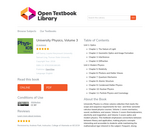Unrestricted Use
CC BY
Rating
0.0 stars

University Physics is a three-volume collection that meets the scope and sequence requirements for two- and three-semester calculus-based physics courses. Volume 1 covers mechanics, sound, oscillations, and waves. Volume 2 covers thermodynamics, electricity and magnetism, and Volume 3 covers optics and modern physics. This textbook emphasizes connections between between theory and application, making physics concepts interesting and accessible to students while maintaining the mathematical rigor inherent in the subject. Frequent, strong examples focus on how to approach a problem, how to work with the equations, and how to check and generalize the result.

Unit 1: Optics

Chapter 1: The Nature of Light
Chapter 2: Geometric Optics and Image Formation
Chapter 3: Interference
Chapter 4: Diffraction
Unit 2: Modern Physics

Chapter 5: Relativity
Chapter 6: Photons and Matter Waves
Chapter 7: Quantum Mechanics
Chapter 8: Atomic Structure
Chapter 9: Condensed Matter Physics
Chapter 10: Nuclear Physics
Chapter 11: Particle Physics and Cosmology

Subject:
Physical Science
Physics
Material Type:
Textbook
Author:
Samuel Ling
Jeff Sanny